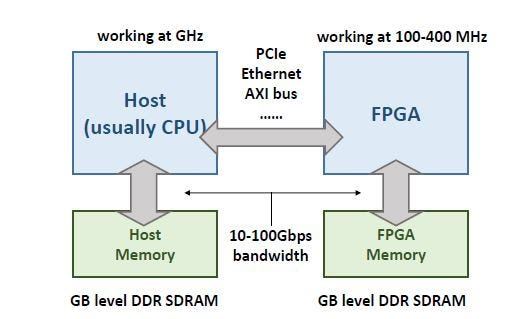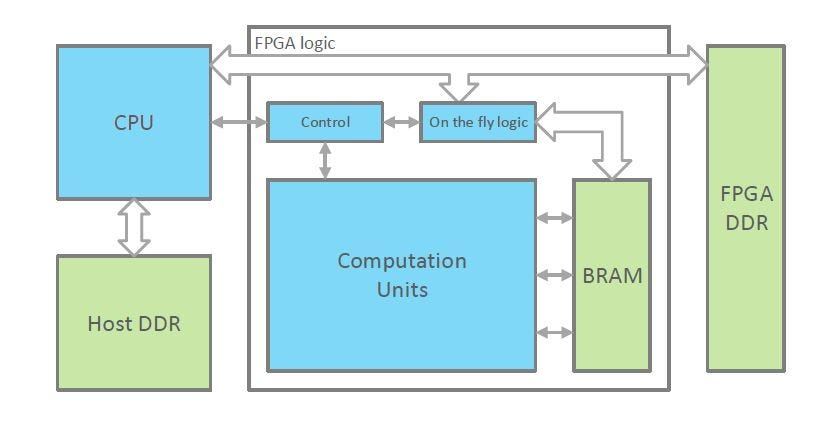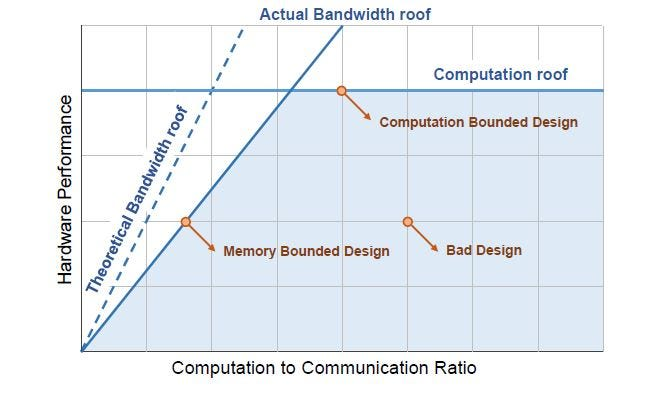# State of the Art FPGAs Design Optimizations

In my previous post, I talked about the general techniques used for the computation and energy efficiency of the neural networks on the available hardware. This post we will cover a breed of hardware called the FPGAs, which has been in the talks some years after the dawn of the neural net era.

## The first question that comes in the mind is, why FPGAs?

So a typical CPU can perform 10–100 GFLOPs per second. A GPU offers up to 10 TFLOP/s peak performance. Thus, CPUs are out of the question as they are badly beaten by the GPUs. But GPUs still have a drawback in terms of energy efficiency and are still general-purpose to have optimization for a specific task.
We all know that ASICs are chips used for specific tasks but the problem with silicon brains is that, once the chip is designed and burnt, it cannot be redesigned again. Here come the FPGAs, reprogrammable ASICs, that’s what I like to call them.
With this re-programmability comes the power to design the chips with high parallelism strategies in mind(which neural nets thrive for badly) and bring the computation load and efficiency compared to GPUs.

## Current problems with FPGAs

• working frequency of the FPGAs is about 100–300MHz, which is much less than CPU and GPU.
• The implementation of neural networks on FPGAs is much harder than on CPUs and GPUs.

Above mentioned are the reason why there is research going on around this breed of silicon, to bring the clock cycles par with the CPU and GPUs and ideally surpass them. There are techniques discussed in the below sections which make mention of hardware optimization techniques for FPGAs.
The current State of the Art neural Network accelerator design estimates at least 10x better energy efficiency the current GPUs.

## Convolution and FC layers

• The convolution and FC layers hold the majority of the proportion of the computation load of a neural network. There are other types of layer to i.e. pooling layer, batch normalization layer, concat etc. but the majority of the weights are concentrated in the convolution and FC layer which involves a multiply and accumulate operations(MACs).

# Overview FPGA based AcceleratorHigh level FPGA based accelerator design
• The system consists of a CPU and an FPGA part. A pure FPGA chip usually works with a host PC/server through PCIe connections. Both the host and the FPGA can work with their own external memory and access each others’ memory through the connection.
• FPGA chips have large on-chip storage units like registers and SRAM, but still too small compared with NN models. Still small to hold of the parameters of a neural net and therefore this gap requires that external memory like DDR SDRAM is needed. The bandwidth and power consumption of DDR limits system performance.

# Design Methodology and Criteria

• In general, the design target of a neural network inference accelerator includes two aspects (i) High Speed(high throughput and low latency) (ii) High energy efficiency.

## Speed

• The on-chip resource of a certain FPGA can be increased by increasing the number of computation units on it, which can be increased by reducing the size of each of the computation units. It can be achieved by reducing the data representation precision i.e. using a 16 bit of 8 bit fixed point representation than floating-point representation in the neural network.
• Secondly, by increasing the working frequency of the chip which can be achieved by carefully designing the chip in the sense that the processed data can be placed very next to the chip. A high chip utilization ratio is ensured by parallelism implementation and efficient memory system. The data access pattern and the data computation ratio also affects if the hardware is fully utilized or not.
Theoretical throughput i.e. number of inferences per second, the formula is given by the following equation :

where OPSact -> the number of operations performed per second at run-time by the accelerator.
W -> the total theoretical workload of the network.
OPSpeak -> maximum number of operations that can be processed per second.
n -> Utilization ratio of the computation units, measured by the average ratio of working computation units in all the computation units during each inference.
f -> working frequency of computation units.
P -> number of computation units.

• Most of the FPGAs- based NN accelerators compute different inputs one by one while some designs process different inputs in parallel. So the latency of the accelerator is expressed as :

where L -> Latency of processing an inference
C -> Concurrency of the accelerator, measured by the number of inferences processed in parallel.
IPS -> Throughput of the system, measured by the number of inferences processed each second.

## Energy Efficiency

• Each operation is performed by a DSP unit which has a certain transistor logic that takes up some electrical voltage to perform a task. Therefore, the energy required to perform the total number of operations in the network is what we consider instead of the number of inferences which was the case with the inference speed.
• If the workload of the network is fixed, increasing the energy efficiency of a neural network accelerator means to reduce the total energy cost.

Eff -> the energy efficieny of the system, measured by the number of operations can be processed within unit energy.
W -> Workload for each inference, measured by the number of operations in the network, mainly addition and multiplication for neural network.
Etotal comprises of the static ram access energy component + dynamic ram access energy component + Static Energy.

We separate the memory access energy into DRAM part and SRAM part. Nx acc can be reduced by quantization, sparsification, efficient on-chip memory system, and scheduling method. Thus these methods help reduce dynamic memory energy.

This article we will focus on the hardware design optimization than on the optimization of the neural network side which involves data quantization, weight reduction i.e. sparsification, weight pruning, weights clustering techniques.

# Hardware Design : Efficient Architecture

## Computation Unit Designs

A smaller computation unit means, more number of computation units can be embedded on the chip which means higher peak performance. A carefully designed computation unit array can increase the working frequency of the system and thus improve the peak performance.

• Low Bit-width Computation unit:
Reducing the bit width, reduces the size of the computation unit, which increases the scope of adding more computation units in the array. More State of the Art designs replace the 32 bit floating point units with fixed points units. 16 bit and 12 bit fixed point representation is being used embed efficient computation unit array on FPGAs. The Binarized NN outperforms CPU and GPU on performance but looses out on the accuracy.
Below is the comparison table for different data representations across different FPGAs chips
• Fast Convolution Method :
The convolution operation on the DSP unit can be faster by using Discrete fourier transformation(DFT). For an FxF filter convolved with KxK filter, DFT converts the (F-K + 1)^2 * ^2 multiplications in the space domain to F² complex multiplications in the frequency domain. For a CONV layer with M input channel and N output channel, MN times of frequency domain multiplications and ¹M + Nº times DFT/IDFT are needed.
The theoretical performance gain from fast convolution depends on the convolution size. Limited by the on-chip resource and the consideration of flexibility, current designs are not choosing large convolution sizes. Existing work point out that up to 4x theoretical performance gain can be achieved by fast convolution with FFT or Winograd with reasonable kernel sizes.
• Frequency Optimization Method :
Latest FPGAs support 700–900 MHz DSP theoretical peak working frequency. But existing design usually works at 100–400 MHz. The working frequency is limited by the routing between on-chip SRAM and DSP units.
Neighbor slices to each DSP unit are used as local RAMs to separate the clock domain.
The prototype design achieves the peak DSP working frequency at 741MHz and 891MHz on FPGA chips of different speed grades. Xilinx has also proposed the CHaiDNN-v2 and xfDNN with this technique and achieves up to 700MHz DSP working frequency. Compared with existing designs for which the frequency is within 300MHz, this technique brings at least 2x peak performance gain.

## Loop Unrolling Strategies

How we loop over the conv layers and FC layers for convolution and multiply-accumulate operations is also a considerable question of research. The inefficient looping strategy can take up a lot of time and bring down the processing efficiency of the system.

Above is the loop unrolling strategy traditionally used. For N number of filters we loop over each of the filter and for each filter channel and for each output map and for each kernel element, we produce the each element of the output feature map by multiplying value of the input feature map with each value of the kernel. Each output map is then added with a bias per channel.
You can go through the looping strategy’s pseudo code for better understanding.

• Choosing Unroll Parameters
The number of parallelized iterations on hardware is called the unroll parameter. Inappropriate unroll parameter selection may lead to a serious hardware underutilization.
Suppose the trip count of the loop is M and the parallelism is m. The utilization ratio of the hardware is the processing an NN layer, the total utilization ratio will be the product of the utilization ration on each of the loop.
Besides the underutilization problem, loop unrolling also affects the datapath and on-chip memory design. Thus loop unrolling strategy is a key feature is for a neural network design.
• Data transfer and On-chip Memory Design
The on-chip memory system should efficiently offer the necessary data to each computation units every cycle.To implement high parallelism, neural network accelerators usually reuse data among a large number of computation units. Simply broadcasting data to different computation units leads to large fan-out and high routing cost and thus reduce the working frequency.
The shared data are transferred from one computation unit to the next in a chain mode. So the data is not broadcasted, and only local connections between different computation units are needed. The drawback is the increase in latency. The loop execution order is scheduled accordingly to cover the latency

## System DesignBlock graph of a typical FPGA-based neural network accelerator system
• The logic part of the whole system is denoted by the blue boxes. e host CPU issues workload or commands to the FPGA logic part and monitors its working status. On the FPGA logic part, a controller is usually implemented to communicate with the host and generates control signals to all the other modules on FPGA. The controller can be an FSM or an instruction decoder.
• The on the fly logic part is implemented for certain designs if the data loaded from external memory needs preprocess. This module can be a data arrangement module, data shifter, FFT module etc.on-chip SRAM
of an FPGA chip is too limited compared with the large NN models. So for common designs, a two-level memory hierarchy is used with DDR and on-chip memory.
• Roofline Model:An example of the roofline model. The shaded part denotes the valid design space given bandwidth and resource limitation.

Computation to communication (CTC) ratio as the x-axis and hardware
performance as the y-axis. CTC is the number of operations that can be executed with a unit size of memory access. Each hardware design can be treated as a point in the figure. So y/x equals to the bandwidth requirement of the design.
The actual bandwidth roof is below the theoretical roof because the achievable bandwidth of DDR depends on the data access pattern. Sequential DDR access achieves much higher bandwidth than random access. The other roof is the computation roof, which is limited by the available resource on FPGA.

• Loop Tiling and Interchange :

The loop unrolling strategies to increase the parallelism while reducing the waste of computation for a certain network. When the loop unrolling strategy is decided, the scheduling of the rest part of the loops decides how the hardware can reuse data with on-chip buffer. This involves loop tiling and loop interchange strategy.
Loop tiling is a higher level of loop unrolling. All the input data of a loop tile will be stored on-chip, and the loop unrolling hardware kernel works on these data. A larger loop tile size means that each tile will be loaded from external memory to on-chip memory fewer times. Loop interchange
strategy decides the processing order of the loop tiles.
The data arrangement in on-chip buffers is controlled through instructions to fit with different feature map sizes. This means the hardware can always fully utilize the on-chip buffer to use the largest tiling size according to on-chip buffer size. This work also proposes the ”back and forth” loop execution order to avoid total on-chip data refresh when an innermost loop finishes.

• Cross-Layer Scheduling:
The external memory access problem by fusing two neighboring layers together to avoid the intermediate result transfer between the two
layers. This strategy helps reduce 95% on-chip data transfer with extra 20% on-chip memory cost.Even software program gains 2 speedup with this scheduling strategy.
• Regularize Data Access Pattern:
Besides increasing CTC, increasing the actual bandwidth roof helps improve the achievable performance with a certain CTC ratio. This is achieved by regularizing the DDR access pattern. The common feature map formats in the external memory include NCHW or CHWN, where N means the batch dimension, C means the channel dimension, H andW means the feature map y and x dimension. Using any of these formats, a feature map tile may be cut into small data blocks stored in discontinuous addresses.

This article is still the tip of the iceberg and just an overview of the what is the State of the Art techniques used in this space to get better computation and energy efficiency on the FPGAs.
Definitely AI on the edge is the next big thing needed to move away from the requirement of the computing on the cloud which is where this field of research holds the most value.

Till next time, keep reading and keep growing :)

Reference : https://arxiv.org/pdf/1712.08934.pdf

--

--